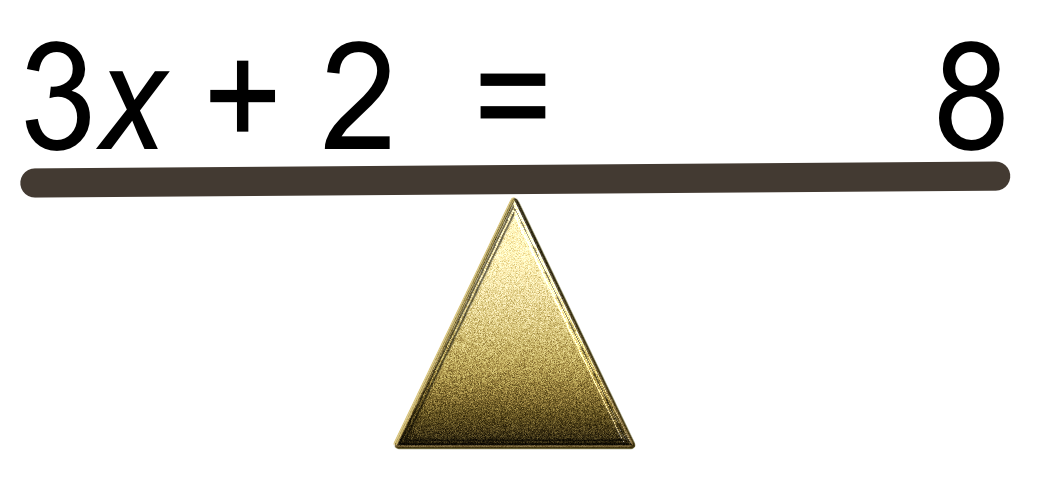Solving Linear Equations

## Solving Linear Equations

A linear equation consists of numbers, and a term that has not been raised to a power. A typical equation would look like 3x + 2 = 8.

The equation may need to be solved, to find the value of the term.

The equation can be seen as a seesaw which must always be balanced, with the equals sign being the fulcrum:The golden rule is if you do something to one side, you must do it to the other. In other words, the equation

The arithmetic is normally carried out by starting with the least powerful BIDMAS operations first, taking additional care where brackets are involved.

You must check your answer is correct by substituting your answer into the original equation, making sure that both sides of the equation give you the same number.

## Example 1

Solve 5y + 7 = 22.

 5y + 7 = 22 Subtract 7 from both sides -7 -7 5y = 15 Divide both sides by 5 ÷5  ÷5 y = 3

Check 5(3)+7=22

Answer: y = 3

## Example 2

Solve 3(a+4)=6.

 3(a + 4) = 6 Multiply out the bracket 3 xx a  3 xx4 3a + 12 = 6 Subtract 12 from both sides -12 -12 3a = -6 Divide both sides by 3 ÷3 ÷3 a = -2

Check: 3((-2) + 4) = 6✔

Answer: a=-2